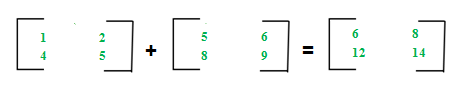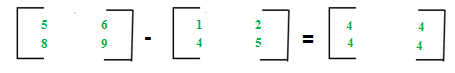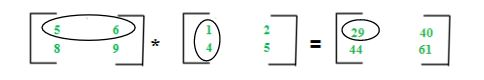Skip to content
Related Articles
Different Operations on Matrices
• Difficulty Level : Easy
• Last Updated : 27 Feb, 2021

For introduction on matrices, you can refer the following article: Matrix Introduction
In this article, we will discuss various operations on matrices and their properties:

Matrices Addition –
The addition of two matrices A m*n and Bm*n gives a matrix Cm*n. The elements of C are sum of corresponding elements in A and B which can be shown as:The algorithm for addition of matrices can be written as:

```for i in 1 to m
for j in 1 to n
cij = aij + bij```

## C++

 `// C++ Program for matrix addition` `#include ``using` `namespace` `std;` `int` `main()``{` `    ``int` `n = 2, m = 2;``    ``int` `a[n][m] = { { 2, 5 }, { 1, 7 } };``    ``int` `b[n][m] = { { 3, 7 }, { 2, 9 } };` `    ``int` `c[n][m];``    ``for` `(``int` `i = 0; i < n; i++)``        ``for` `(``int` `j = 0; j < n; j++) {``            ``c[i][j] = a[i][j] + b[i][j];``        ``}` `    ``for` `(``int` `i = 0; i < n; i++) {``        ``for` `(``int` `j = 0; j < n; j++)``            ``cout << c[i][j] << ``" "``;``        ``cout << endl;``    ``}``}`

Output:

```5 12
3 16 ```

Time Complexity: O(n * m)

Auxiliary Space: O(n * m)
Key points:

• Addition of matrices is commutative which means A+B = B+A
• Addition of matrices is associative which means A+(B+C) = (A+B)+C
• The order of matrices A, B and A+B is always same
• If order of A and B is different, A+B can’t be computed
• The complexity of addition operation is O(m*n) where m*n is order of matrices

Matrices Subtraction –
The subtraction of two matrices Am*n and Bm*n gives a matrix Cm*n. The elements of C are difference of corresponding elements in A and B which can be represented as:The algorithm for subtraction of matrices can be written as:

```for i in 1 to m
for j in 1 to n
cij = aij-bij```

## C++

 `// C++ Program for matrix substraction` `#include ``using` `namespace` `std;` `int` `main()``{` `    ``int` `n = 2, m = 2;``    ``int` `a[n][m] = { { 2, 5 }, { 1, 7 } };``    ``int` `b[n][m] = { { 3, 7 }, { 2, 9 } };` `    ``int` `c[n][m];``    ``for` `(``int` `i = 0; i < n; i++)``        ``for` `(``int` `j = 0; j < n; j++) {``            ``c[i][j] = a[i][j] - b[i][j];``        ``}` `    ``for` `(``int` `i = 0; i < n; i++) {``        ``for` `(``int` `j = 0; j < n; j++)``            ``cout << c[i][j] << ``" "``;``        ``cout << endl;``    ``}``}`

Output:

```-1 -2
-1 -2 ```

Key points:

• Subtraction of matrices is non-commutative which means A-B ≠ B-A
• Subtraction of matrices is non-associative which means A-(B-C) ≠ (A-B)-C
• The order of matrices A, B and A-B is always same
• If order of A and B is different, A-B can’t be computed
• The complexity of subtraction operation is O(m*n) where m*n is order of matrices

Matrices Multiplication –
The multiplication of two matrices Am*n and Bn*p gives a matrix Cm*p. It means number of columns in A must be equal to number of rows in B to calculate C=A*B. To calculate element c11, multiply elements of 1st row of A with 1st column of B and add them (5*1+6*4) which can be shown as:The algorithm for multiplication of matrices A with order m*n and B with order n*p can be written as:

```for i in 1 to m
for j in 1 to p
cij = 0
for k in 1 to n
cij += aik*bkj```

## C++

 `// C++ Program for matrix Multiplication` `#include ``using` `namespace` `std;` `int` `main()``{` `    ``int` `n = 2, m = 2;``    ``int` `a[n][m] = { { 2, 5 }, { 1, 7 } };``    ``int` `b[n][m] = { { 3, 7 }, { 2, 9 } };` `    ``int` `c[n][m];``    ``int` `i, j, k;``    ``for` `(i = 0; i < n; i++)``    ``{``        ``for` `(j = 0; j < n; j++)``        ``{``            ``c[i][j] = 0;``            ``for` `(k = 0; k < n; k++)``                ``c[i][j] += a[i][k] * b[k][j];``        ``}``    ``}` `    ``for` `(``int` `i = 0; i < n; i++)``    ``{``        ``for` `(``int` `j = 0; j < n; j++)``            ``cout << c[i][j] << ``" "``;``        ``cout << endl;``    ``}``}`

Output:

```16 59
17 70```

Key points:

• Multiplication of matrices is non-commutative which means A*B ≠ B*A
• Multiplication of matrices is associative which means A*(B*C) = (A*B)*C
• For computing A*B, the number of columns in A must be equal to number of rows in B
• Existence of A*B does not imply existence of B*A
• The complexity of multiplication operation (A*B) is O(m*n*p) where m*n and n*p are order of A and B respectively
• The order of matrix C computed as A*B is m*p where m*n and n*p are order of A and B respectively

Attention reader! Don’t stop learning now. Get hold of all the important DSA concepts with the DSA Self Paced Course at a student-friendly price and become industry ready.  To complete your preparation from learning a language to DS Algo and many more,  please refer Complete Interview Preparation Course.

In case you wish to attend live classes with industry experts, please refer DSA Live Classes

My Personal Notes arrow_drop_up